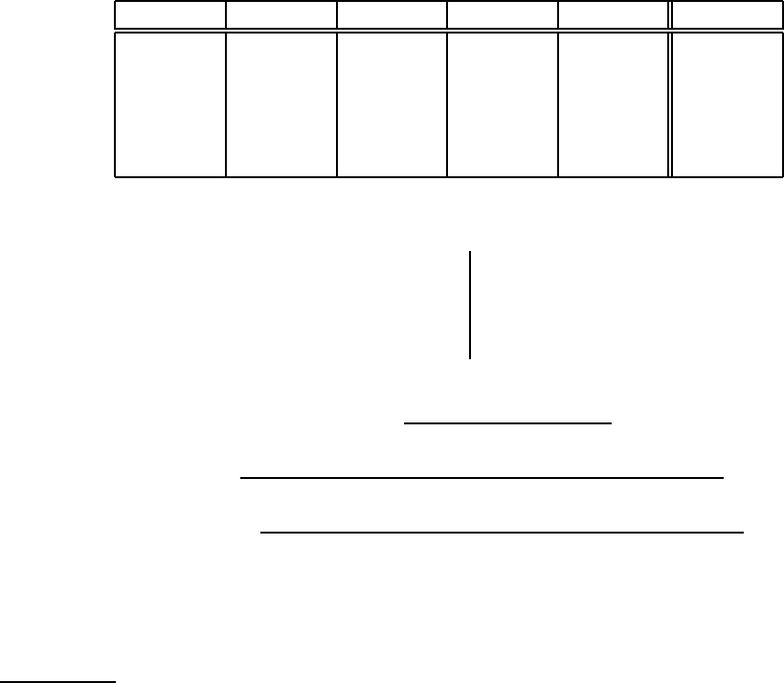Study Guides (380,000)
US (220,000)
UCLA (3,000)
MATH (300)
All (20)
Final

MATH 33AH Lecture Notes - Lecture 20: Empty Set, Ab1Exam

Department
Mathematics
Course Code
MATH 33AH
Professor
All
Study Guide
Final

This preview shows pages 1-2. to view the full 7 pages of the document.MATH 33A LECTURE 3
PRACTICE MIDTERM I
tions will receive little or no credit (except on multiple-choice problems). Please call one
of the proctors if you have any questions about a problem. No calculators, computers,
PDAs, cell phones, or other devices will be permitted. If you have a question about the
grading or believe that a problem has been graded incorrectly, you must bring it to the
attention of your professor within 2 weeks of the exam.
#1 #2 #3 #4 #5 Total
TA name (circle):
Your section meets (circle): Ioannis Lagkas-Nikolos
Tuesday Thursday Jaehoon Lee
Austin Christian
Student ID:
Name:
Signature:
By signing above I certify that I am the person
2016 UC Regents/Dimitri Shlyakhtenko. No part of this exam may be reproduced by any
means, including electronically.
1

Only pages 1-2 are available for preview. Some parts have been intentionally blurred.MATH 33A LECTURE 3 PRACTICE MIDTERM I 2
Problem 1. (True/False, 1 pt each) Mark your answers by ﬁlling in the appropriate box
next to each question (no explanations are necessary on this problem).
(a T F ) There exists a 2 ×2 matrix Aso that A 6=0, A · A 6=0 but A · A · A =0. NO.
Since Ais 2 ×2, ker Ais a subspace of R2and can have dimension 0, 1 or 2. If
dim ker A=2, A=0; but we are given that A 6=0. So ker Acan be dimension 0
or dim 1. If ker Ahad dimension 0, the nullity of Awould be zero, so the rank of A
would be 2 by the rank-nullity theorem. So Awould invertible, but then A3would
also be invertible and so could not be zero. So ker Amust be one-dimensional.
By rank-nullity also im Ais then also one-dimensional.
Let’s say that {v} is a basis for im A. Since Av is in the image of A,Av must be a
multiple of v:Av =αv.
If α 6=0, we would get that A3v=A · A · Av =A · Aαv =2v=α3v 6=0,
contradicting A3=0. So αmust be zero.
If α=0, then Av =0. Let wbe any vector. Then Aw is in the image of A, thus
a multiple of v. Then A·Aw must be a mutliple of Av, which is zero. So A2=0,
again a contradiction. Thus such an Acannot exist.
(b T F ) There are two 2 ×2 matrices A,Bso that AB 6=BA.YES. Take for example A=
0 0
0 1
,B=
0 1
1 0
. Then Ae1=0, Ae2=e2,Be1=e2so ABe1=Ae2=e2
but BAe1=0 because Ae1=0. So ABe16=BAe1so AB 6=BA.
(c T F ) If Ais an n × n matrix with rank A=3 then the system of equations Ax =0
always has exactly one solution. NO. The solution would be unique exactly when
rank A=n; so if n > 3 there would be more than one solution.
(d T F ) If Ais an n×m matrix and im A=Rn, then n ≤ m.YES. The image is spanned
by mvectors Ae1,...,Aem, so by assumption Rnis spanned by these mvectors.
Since dim Rn=nit must be that m ≥ n.